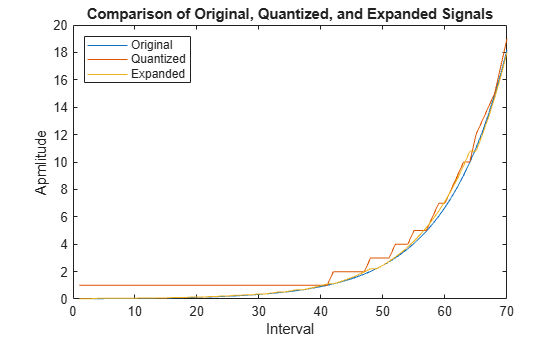## Compand a Signal

In certain applications, such as speech processing, it is common to use a logarithm computation, called a compressor, before quantizing. The inverse operation of a compressor is called an expander. The combination of a compressor and expander is called a compander.

The `compand` function supports two kinds of companders: µ-law and A-law companders. Its reference page lists both compressor laws.

### Quantize and Compand an Exponential Signal

Quantize an exponential signal with and without companding and compare the mean square distortions.

Set the μ-law parameter `Mu`.

`Mu = 255;`

Create an exponential signal and find its maximum value.

```sig = exp(-4:0.1:4); V = max(sig);```

Quantize the signal using equal-length intervals. Set the `partition` and `codebook` arguments assuming six bit quantization.

```partition = 0:2^6-1; codebook = 0:2^6; [~,~,distor] = quantiz(sig,partition,codebook);```

Compress the signal using the `compand` function. Apply quantization and expand the quantized signal. Calculate the mean square distortion.

```compsig = compand(sig,Mu,V,'mu/compressor'); [~,quants] = quantiz(compsig,partition,codebook); newsig = compand(quants,Mu,max(quants),'mu/expander'); distor2 = sum((newsig-sig).^2)/length(sig);```

Compare the mean square distortions. The output shows that the distortion is smaller when companding is used. This is because equal-length intervals are well suited to the logarithm of `sig` but not well suited to `sig` itself.

`[distor, distor2]`
```ans = 1×2 0.5348 0.0397 ```

Plot the signal and its companded version.

```plot([sig' compsig']) legend('Original','Companded','location','nw')```